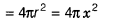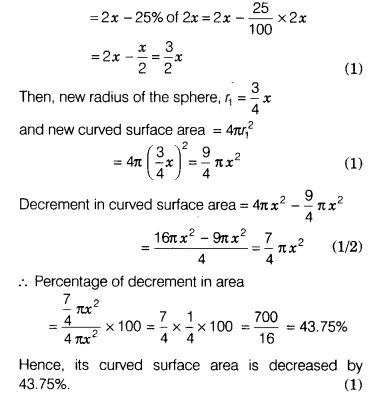# The diameter of a sphere is decreased by 25%.By what per cent does its curved surface area

The diameter of a sphere is decreased by 25%. By what per cent does its curved surface area decrease?

Let the original diameter of sphere be 2x. Then, original radius of the sphere, r = x
Original curved surface area of sphereNow, diameter of a sphere is decreased by 25%, so new diameter of sphere